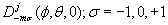NONLINEAR PHENOMENA IN COMPLEX SYSTEMS
An Interdisciplinary Journal

2010, Vol.13, No.4, pp.352-367

On the Wave Functions and Energy Spectrum for a Spin 1 Particle in an External Coulomb Field.
V. V. Kisel, E.M. Ovsiyuk, and V. M. Red'kov

Quantum-mechanical system - spin 1 particle in an external Coulomb field is studied on the base of the matrix Duffin - Kemmer - Petiau formalism with the use of the tetrad technique. Separation of the variables is performed with the help of Wigner functions; j and m stand for quantum numbers determining the square and the third projection of the total angular momentum of the vector particle. With the help of parity operator, the radial 10-equation system is divided into two subsystem of 4 and 6 equations that correspond to parity P=(-1)j+1 and P= (-1)j respectively. The system of 4 equation is reduced to a second order differential equation which coincides with that arising in the case of a scalar particle in a Coulomb potential. It is shown that the 6-equation system reduces to two different second order differential equations for a "main" function. One main equation reduces to a confluent Heun equation and provides us with the energy spectrum. Another main equation is a more complex one, and solutions for it remain unknown.
Key words: quantum mechanics, spin 1 particle, bound states, Coulomb field

Full text:  Acrobat PDF  (549KB)

Copyright © Nonlinear Phenomena in Complex Systems. Last updated: March 10, 2011